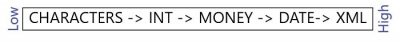# Data Type ConversionWhen combining Data Types, it is important to note that there is a data type precedence used to determine how they are converted and combined. Data types with a lower precedence are implicitly converted to the data type with the higher precedence.

If a data type of a higher precedence is needed to be converted to a data type of a lower precedence, then an explicit conversion must be made using either the CAST or CONVERT functions.

``````--Implicit Conversion
SELECT 42 + 11 + '25' AS 'Result'``````

Next, we will try where we have actual text that cannot be converted into an integer. Since a character data type has a lower precedence than an integer and SQL cannot perform the implicit conversion and will display the following result.

``````--Conversion Error
SELECT 42 + 11 + 'Total' AS 'Result'``````

So, to combine the text to the integer, the integer will have to be explicitly converted using either the CAST or CONVERT functions. The main difference between the CAST and CONVERT functions is that CAST is an ISO standard whereas CONVERT is specific to Transact-SQL and can also use styles for numbers and dates. In both cases, the following result will be displayed.

``````--Explicit Conversion using CAST
SELECT CAST(42 + 11 as char(2)) + ' Total' AS 'Result'

--Explicit Conversion using CONVERT
SELECT CONVERT(char(2), 42 + 11) + ' Total' AS 'Result'``````

Here is an excellent conversion chart that will help in determining which data type can or cannot be converted.

#### Be the first to commenton "Data Type Conversion"

This site uses Akismet to reduce spam. Learn how your comment data is processed.